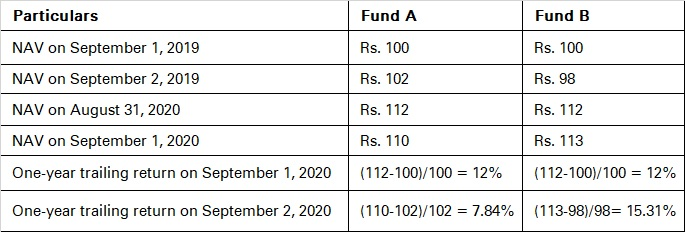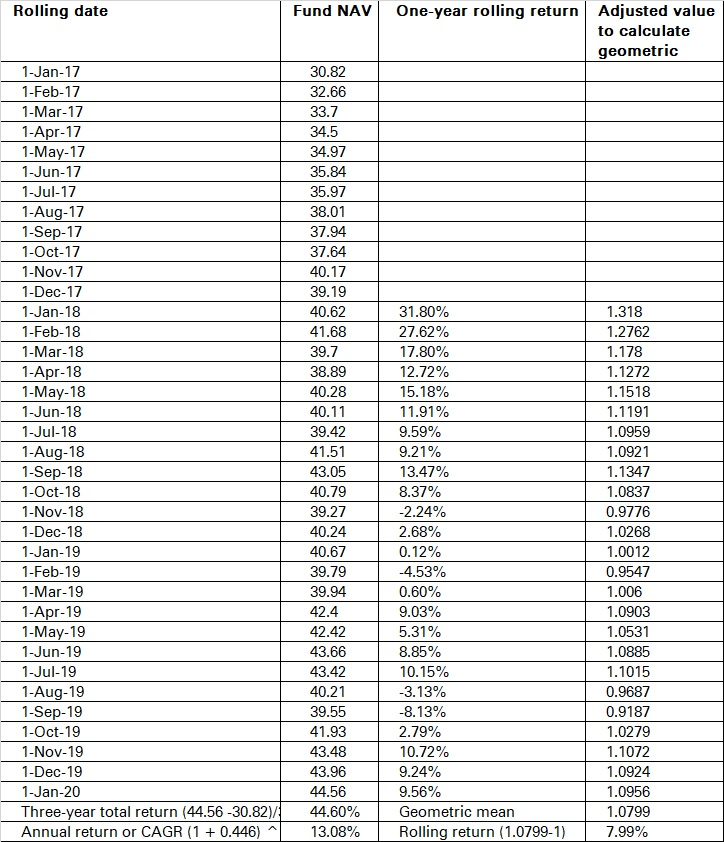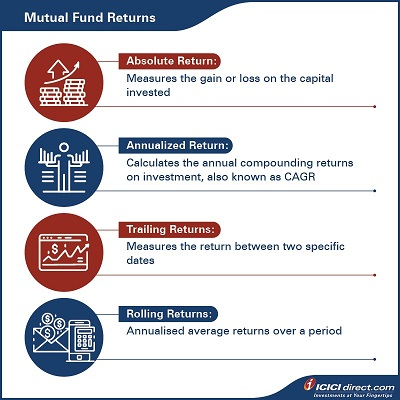If number crunching is not your thing, it is time to make peace. There are some numbers you cannot ignore. Over a period, you may invest in multiple mutual fund schemes. If you are serious about creating wealth, you need to review the performance of these schemes regularly. Not all mutual funds generate the same return. You need to know outperformers and underperformers.

Often, investors depend on distributors, advisors, SIP calculators or similar tools to know the returns on their investments. Sometimes it becomes confusing which types of returns are showing me the right picture.  We will discuss different types of returns and how to calculate them in this chapter.  It will help you to calculate the return yourself with ease.

## Types of returns

Before we jump into return calculations, let us take a look at the different types of returns:

## 1. Absolute return:

Absolute return measures the gain or loss of a mutual fund as a percentage of the capital invested. It calculates return irrespective of time.

Absolute returns =  (Selling Price – Cost Price) x 100
Cost Price

Let’s say you have Rs. 10,000 in a mutual fund. After three years, it grows to Rs.15,000. Your absolute return will be (Rs. 15,000 – Rs. 10,000)*100/Rs. 10,000 = 0.5 or 0.5*100 = 50%

 Did you know?  Absolute return is also known as holding period return. This is normally used for lumpsum investments.

### 2. Annualized return:

Also called Compounded Annual Growth Rate (CAGR), annualized return is the geometric average of an investment's earnings for its holding period.

Annualized return = (Today’s value/initial investment)   1/n – 1

where n = holding period in years

If we take the same example as above, annualized return will be:

Annualized return = (Rs. 15,000/Rs. 10,000) 1/3 – 1 = 0.1447

or 0.1447*100 = 14.47%

### 3. Real rate of return:

The real rate of return is the inflation-adjusted return of an investment. Inflation eats into your returns. Your money has to grow at a faster rate than inflation to grow.  The real rate shows the real increase of your investment in terms of purchasing power.
• For a real growth, investment return should be more than inflation.

Real return = {(1+Investment return) / (1+Inflation rate)}-1

For example, if you assume inflation is 6% and investment return is 10%, real return can be calculated as:

Real return = [(1+0.1)/(1+0.06)] -1 = 0.0377 or 0.0377*100 = 3.77%

### 4. Post-tax return:

This is the return that you get after you pay the tax applicable.

Post-tax return = Investment return x (1 – Tax rate)

Let’s suppose tax rate is 30% and your investment return is 10%. Then, your post-tax return will be:

Post-tax return = 10% * (1-0.3) = 0.07 or 0.07*100 = 7%

### 5. Tax-adjusted real rate of return:

Every mutual fund investment attracts capital gains tax, be it short-term or long-term. Tax-adjusted real rate of return calculates the real increase in purchasing power after tax.

Tax-adjusted real rate of return = {(1+ Tax adjusted rate of return)/(1+inflation rate)}-1

If we take the above example where post-tax return is 7% and inflation rate is 6%, then

Tax-adjusted real rate of return = [(1+0.07)/(1+0.06)]-1 = 0.0094 or 0.0094*100 = 0.94%

It is more credible to look at your real returns on any investments.

### 6. Trailing and Rolling returns:

Trailing return is point to point return. This return depends on the date of entry and exit and can have large variations. If you are considering a one-year trailing return on September 1, 2020, it means you consider the return for a year, i.e. from September 1, 2019 to August 31, 2020. Let us understand this with an example:The performance of Fund A and B is the same if we look at the trailing return on September 1, 2020. The performance of Fund B is better than A if you look at the data on September 2, 2020. To calculate the trailing return on September 2, 2020, you need to consider the time frame from September 2, 2019 to September 1, 2020.This can be confusing. A better measure is to look at rolling returns.

Rolling return focuses on the holding period return instead of calculating the return from one date to another. To calculate rolling returns, you need to decide the total period of return calculation and the interval. If you want to calculate five-year rolling returns with a monthly interval, then you will need to calculate the return of the last five years every month and calculate an average. The interval frequency depends on the period you choose for the return calculation.

• For three months rolling returns, daily intervals work well.
• For a one-year period, weekly data is okay.
• For more than three years, monthly data works best.

Let us understand this with an example.  You want to calculate the one-year rolling return with a monthly interval and have taken three years i.e. from January 1, 2017 to January 1, 2020. You take the monthly NAV and calculate yearly returns from January 2018 onward. After this, you can use the geometric mean formula on Microsoft Excel (GEOMEAN) and calculate rolling returns. The rolling return in this case is 7.99% p.a.

If you calculate the CAGR from January 1, 2017 to January 1, 2020, it comes to 13.08%. This return can be earned only if you have entered and exited on these dates only.A good way to check the performance of a fund in the long-term is to look at rolling returns. If the difference between rolling return and trailing return is not large, it means that the fund’s performance is consistent.

If you are investing through SIPs, you need to focus on rolling returns because you would expect the fund to perform consistently.

These are the most common ways of calculating mutual fund returns. You can easily estimate how well your investment is doing and alter your portfolio, if required.#### Summary

• There are four different returns you can look at to evaluate your mutual fund’s performance:
• Absolute return
• Annualized return
• Trailing returns
• Rolling returns
• Absolute returns = (Selling Price – Cost Price) x 100

Cost Price

• Annualized return = (Today’s value/initial investment) 1/n – 1
• A good way to check the performance of a fund in the long-term is to look at rolling returns.
• Trailing returns are the past returns of the mutual fund till date for specific years.

These are manual ways to calculate returns on your investments. Of course, there is an easier way: via Microsoft Excel! In the final chapter, we will look at the ways in which you can calculate your mutual fund investment returns using Excel formulae.

Disclaimer:

ICICI Securities Ltd. ( I-Sec). Registered office of I-Sec is at ICICI Securities Ltd. ICICI Venture House, Appasaheb Marathe Marg, Prabhadevi, Mumbai - 400 025, India, Tel No : 022 - 6807 7100. I-Sec acts as a Composite Corporate agent having registration number –CA0113. PFRDA registration numbers:  POP no -05092018. AMFI Regn. No.: ARN-0845. We are distributors for Mutual funds and National Pension Scheme (NPS). Mutual Fund Investments are subject to market risks, read all scheme related documents carefully. Please note, Mutual Fund and NPS related services are not Exchange traded products and I-Sec is just acting as distributor to solicit these products. Please note, Insurance related services are not Exchange traded products and I-Sec is acting as a corporate agent to solicit these products. All disputes with respect to the distribution activity, would not have access to Exchange investor redressal forum or Arbitration mechanism.  The contents herein above shall not be considered as an invitation or persuasion to trade or invest.  I-Sec and affiliates accept no liabilities for any loss or damage of any kind arising out of any actions taken in reliance thereon. Investments in securities market are subject to market risks, read all the related documents carefully before investing. The contents herein mentioned are solely for informational and educational purpose.